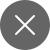# 4 Types of Machine Learning

3+

## What is Machine Learning?

It’s the property of computers to learn and improvise from previous experiences without being externally programmed.

It’s of 4 types:

• Supervised Learning model
• Unsupervised Learning model
• Reinforcement model
• Semi-Supervised model

## Types of Machine Learning

### SUPERVISED LEARNING

• It happens under supervision.
• In Supervised machine learning, the model forms a prediction using labeled data.
• Labeled data refers to the dataset having both input and output parameters.
• After this, the model is provided with the unlabeled data.
• The model makes its (unlabeled data) prediction using the prediction made with labeled data.
• Example: Suppose you are given a basket of different vegetables as unlabeled data. Under Supervised algorithm,

you will make the machine learn about the data and make it labeled.

(Example- if the shape of the vegetable is rounded, is red, and has a green crown at its top, label it as – TOMATO) etc.

After training, your machine is given a vegetable to identify its name and because it is trained, it will analyze the shape and color of the vegetable and tell its name.### How to train your system?

The whole dataset is divided into testing data and training data in the ratio of 20:80 but the model learns from training data only. During training, input data is made familiar with 80% of the training data. Different machine learning algorithms are used to build a model. After the model is built using training data, it is ready to be treated with testing data. Here, the input is treated with 20% of the testing data after which the model makes predictions and calculates various results.

Types of Supervised Learning:

1. Regression: It is a type of Supervised learning where the target variable/output is a continuous numerical value. The aim of using the Regression model is to predict a value much closer to the actual value so that there is less difference means a small error. A small error can assure accuracy in our model.
1. Classification: It is a type of Supervised learning where the target variable/output is a categorical value i.e. 0 or 1. 0 can mean no and 1 can mean yes. The aim of using the Classification model is to predict a discrete value from a particular class and then calculate accuracy on that basis.

Examples of Supervised Learning:

1. Decision Trees
2. Random Forest
3. Linear Regression
4. Gaussian Naïve Bayes

1. It learns from experience which means better after each performance.
2. It is mostly used for real-world problems
3. It allows data collection and production of output learning from experience.

1. Difficult to handle large datasets.
2. It’s a time-consuming process.

## Unsupervised Learning

1. It’s the compliment of Supervised Learning.
2. There are no target variables involved.
3. It works on unlabeled data.
4. This model identifies if some patterns are existing in data.
5. It is a type of machine learning that is deployed to find patterns in unlabeled data.
6. Example- Population dataset (State, number of family members, date, income, senior citizens) – there are no target variables involved.
7. In such types of data, groups can be based on any pattern.Types of Unsupervised Machine Learning:

1. Clustering: In this type of machine learning, a set of objects are grouped with similar data. The aim of doing Clustering is to easily understand and manipulate a given data.
1. Association: In this type of machine learning, relations between large datasets are discovered. It is a kind of rule-learning problem. The aim of using this is to find patterns in large portions of data.

Examples of Unsupervised Learning:

1. K-Means Clustering
2. Hierarchical Clustering
3. BIRCH

1. Labeled data is not required.
2. It has a fast classification process.
3. It saves time.

1. It only performs the classification tasks.
2. Due to outliers, results are not constant.
3. It does not estimate the result of new data.

## Reinforcement Learning Model

• It’s a type of machine learning used to make certain decisions to increase the rewards.
• With every processing, the model keeps on improving itself to learn a specific pattern.
•  The algorithm used in Reinforcement learning is issue-specific, like – self-driving cars.
• These models learn from mistakes.

Process for Reinforcement learning deployment-

1. An agent observes the inputs in data.
2. The agent performs an action based on the reading.
3. After the performance, the agent receives a reward and reinforces the model which is after deployment stored in the state-action category.

Algorithms used in Reinforcement

• Q-learning
• SARSA(State–action–reward–state–action)
• Deep Q Network etc

## Semi-Supervised Learning

• As the name suggests, it is a technique using both supervised algorithms and unsupervised algorithms.
• It uses a large amount of unlabeled data with a tiny amount of labeled data when training a dataset.
• It is mostly used with image datasets.
• It can be used to predict labels and then providing them with supervised learning techniques.

Examples:

• Text Document Classifier
• Speech analyst etc.3+

### 3 Responses

1. December 20, 2020

[…] always have to validate your machine learning model. In machine learning, you cannot just fit the model on the training data and say it will work […]

0
2. January 1, 2021

[…] Machine Learning Algorithms: Algorithm is a technique we use to predict the results by training a set of data. In Machine learning, there are numerous algorithms that we use frequently based on which type of output we need. There are three types of algorithms in ML are Supervised Learning, Unsupervised Learning, and Reinforcement Learning. This article helps you to understand these algorithms in clear Machine learning models. […]

0
3. January 24, 2021

[…] Unsupervised Learning […]

0